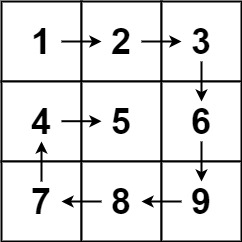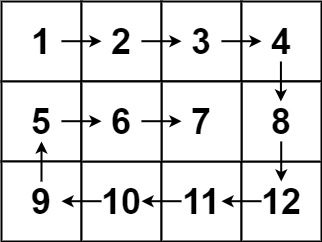0054. 螺旋矩阵

# 0054. 螺旋矩阵#

• 标签：数组
• 难度：中等

## 题目大意 #

• $m == matrix.length$。
• $n == matrix[i].length$。
• $1 \le m, n \le 10$。
• $-100 \le matrix[i][j] \le 100$。

 1 2  输入：matrix = [[1,2,3],[4,5,6],[7,8,9]] 输出：[1,2,3,6,9,8,7,4,5]1 2  输入：matrix = [[1,2,3,4],[5,6,7,8],[9,10,11,12]] 输出：[1,2,3,4,8,12,11,10,9,5,6,7]## 解题思路 #

### 思路 1：模拟 #

1. 使用数组 ans 存储答案。然后定义一下上、下、左、右的边界。
2. 然后按照逆时针的顺序从边界上依次访问元素。
3. 当访问完当前边界之后，要更新一下边界位置，缩小范围，方便下一轮进行访问。
4. 最后返回答案数组 ans

### 思路 1：代码 #

  1 2 3 4 5 6 7 8 9 10 11 12 13 14 15 16 17 18 19 20 21 22 23 24 25 26  class Solution: def spiralOrder(self, matrix: List[List[int]]) -> List[int]: up, down, left, right = 0, len(matrix)-1, 0, len(matrix)-1 ans = [] while True: for i in range(left, right + 1): ans.append(matrix[up][i]) up += 1 if up > down: break for i in range(up, down + 1): ans.append(matrix[i][right]) right -= 1 if right < left: break for i in range(right, left - 1, -1): ans.append(matrix[down][i]) down -= 1 if down < up: break for i in range(down, up - 1, -1): ans.append(matrix[i][left]) left += 1 if left > right: break return ans 

### 思路 1：复杂度分析 #

• 时间复杂度：$O(m * n)$。其中 $m$、$n$ 分别为二维矩阵的行数和列数。
• 空间复杂度：$O(m * n)$。如果算上答案数组的空间占用，则空间复杂度为 $O(m * n)$。不算上则空间复杂度为 $O(1)$。